JAJSCT3A November   2016  – January 2017

PRODUCTION DATA.

1. 1特長
2. 2アプリケーション
3. 3概要
4. 4改訂履歴
5. 5Specifications
6. 6デバイスおよびドキュメントのサポート
7. 7メカニカル、パッケージ、および注文情報

• DQJ|8

## 5 Specifications

### 5.1 Electrical Characteristics

TA = 25°C (unless otherwise stated)
PARAMETER TEST CONDITIONS MIN TYP MAX UNIT
STATIC CHARACTERISTICS
BVDSS Drain-to-source voltage VGS = 0 V, ID = 250 μA 40 V
IDSS Drain-to-source leakage current VGS = 0 V, VDS = 32 V 1 μA
IGSS Gate-to-source leakage current VDS = 0 V, VGS = 20 V 100 nA
VGS(th) Gate-to-source threshold voltage VDS = VGS, ID = 250 μA 1.5 1.8 2.4 V
RDS(on) Drain-to-source on resistance VGS = 4.5 V, ID = 14 A 6.0 7.9
VGS = 10 V, ID = 14 A 4.1 4.9
gfs Transconductance VDS = 4 V, ID = 14 A 59 S
DYNAMIC CHARACTERISTICS
Ciss Input capacitance VGS = 0 V, VDS = 20 V, ƒ = 1 MHz 2060 2680 pF
Coss Output capacitance 205 267 pF
Crss Reverse transfer capacitance 106 138 pF
RG Series gate resistance 1.3 2.6 Ω
Qg Gate charge total (4.5 V) VDS = 20 V, ID = 14 A 14 18 nC
Qg Gate charge total (10 V) 29 38 nC
Qgd Gate charge gate-to-drain 5.0 nC
Qgs Gate charge gate-to-source 6.0 nC
Qg(th) Gate charge at Vth 3.4 nC
Qoss Output charge VDS = 20 V, VGS = 0 V 9.2 nC
td(on) Turnon delay time VDS = 20 V, VGS = 10 V,
IDS = 14 A, RG = 0 Ω
13 ns
tr Rise time 22 ns
td(off) Turnoff delay time 14 ns
tf Fall time 6 ns
DIODE CHARACTERISTICS
VSD Diode forward voltage ISD = 14 A, VGS = 0 V 0.8 1.0 V
Qrr Reverse recovery charge VDS= 20 V, IF = 14 A,
di/dt = 300 A/μs
8.5 nC
trr Reverse recovery time 9 ns

### 5.2 Thermal Information

TA = 25°C (unless otherwise stated)
THERMAL METRIC MIN TYP MAX UNIT
RθJC Junction-to-case thermal resistance(1) 1.7 °C/W
RθJA Junction-to-ambient thermal resistance(1)(2) 50
RθJC is determined with the device mounted on a 1-in2 (6.45-cm2), 2-oz (0.071-mm) thick Cu pad on a 1.5-in × 1.5-in
(3.81-cm × 3.81-cm), 0.06-in (1.52-mm) thick FR4 PCB. RθJC is specified by design, whereas RθJA is determined by the user’s board design.
Device mounted on FR4 material with 1-in2 (6.45-cm2), 2-oz (0.071-mm) thick Cu.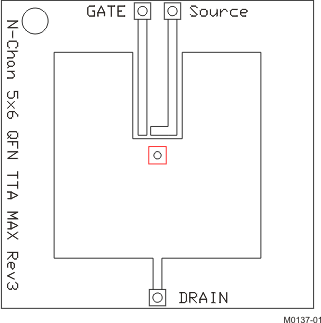Max RθJA = 50°C/W when mounted on 1 in2 (6.45 cm2) of 2-oz (0.071-mm) thick Cu.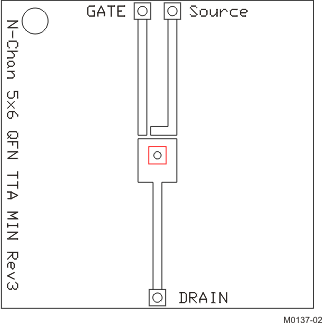Max RθJA = 125°C/W when mounted on a minimum pad area of 2-oz (0.071-mm) thick Cu.

### 5.3 Typical MOSFET Characteristics

TA = 25°C (unless otherwise stated)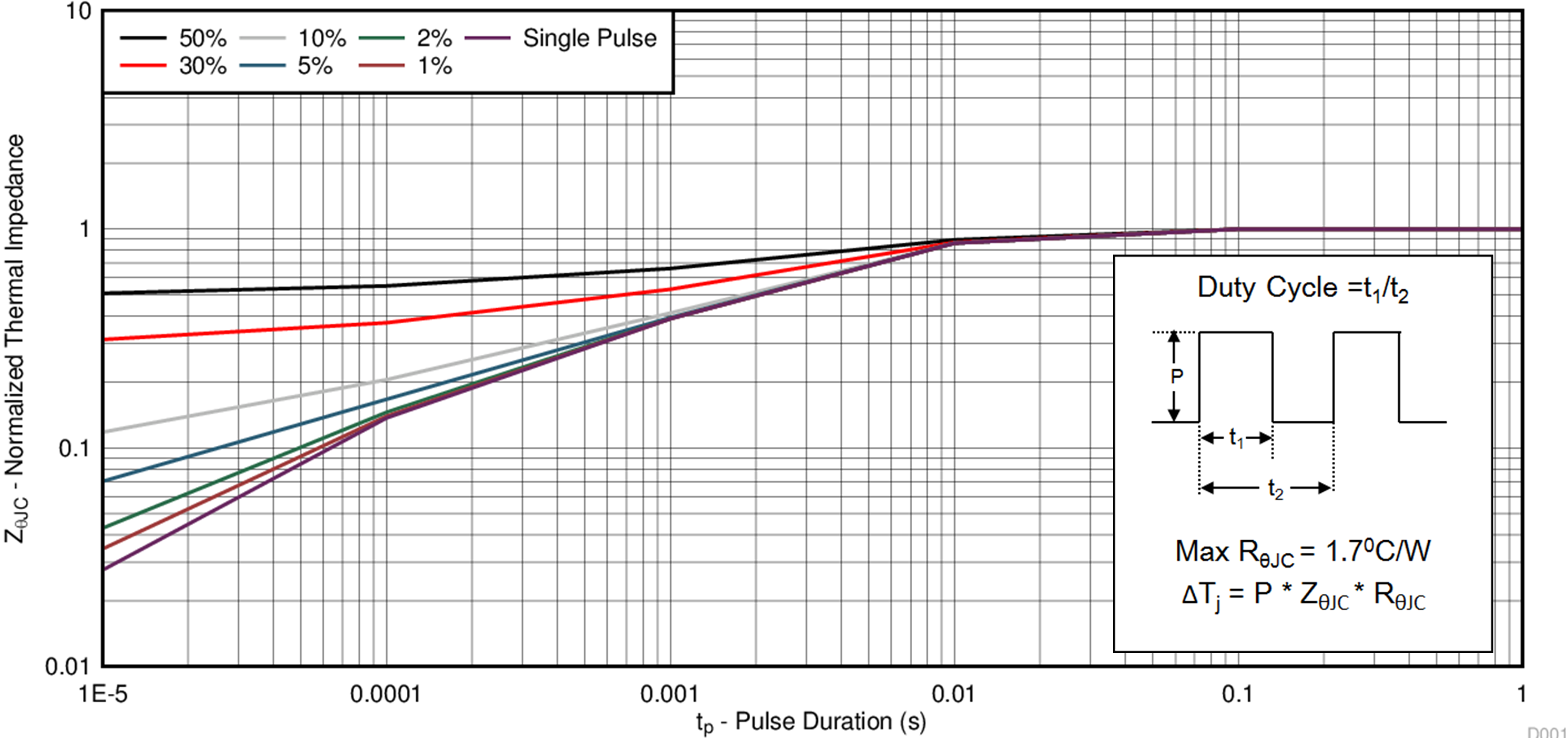Figure 1. Transient Thermal Impedance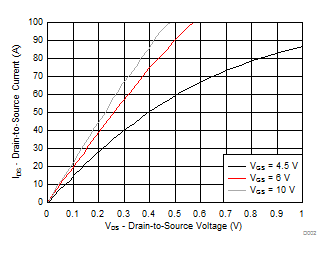Figure 2. Saturation Characteristics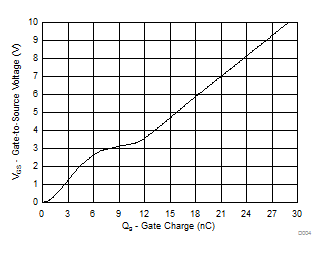ID = 14 A, VDS = 20 V
Figure 4. Gate Charge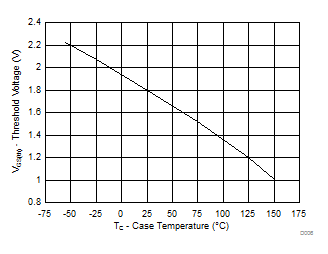ID = 250 µA
Figure 6. Threshold Voltage vs Temperature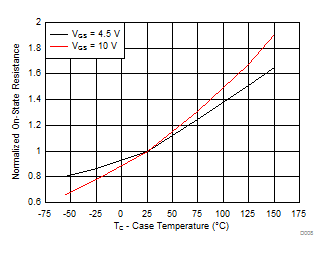ID = 14 A
Figure 8. Normalized On-State Resistance vs Temperature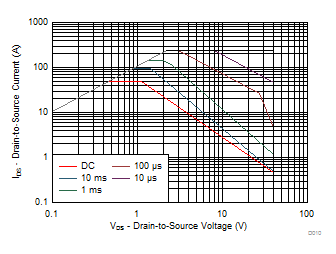Single pulse, max RθJC= 1.7°C/W
Figure 10. Maximum Safe Operating Area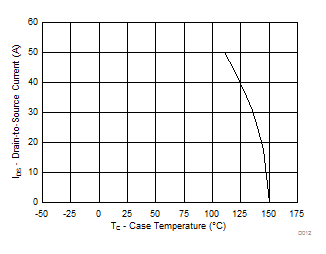Max RθJC= 1.7°C/W
Figure 12. Maximum Drain Current vs Temperature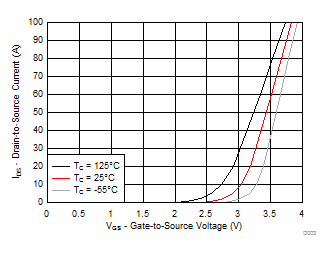VDS = 5 V
Figure 3. Transfer Characteristics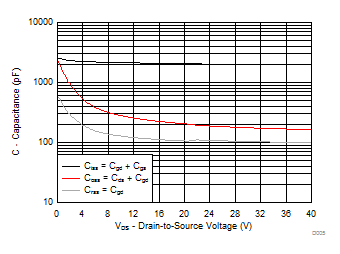Figure 5. Capacitance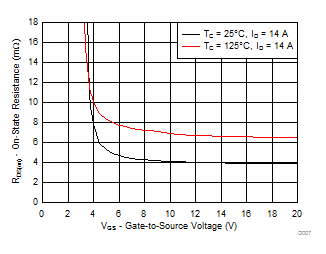Figure 7. On-State Resistance vs Gate-to-Source Voltage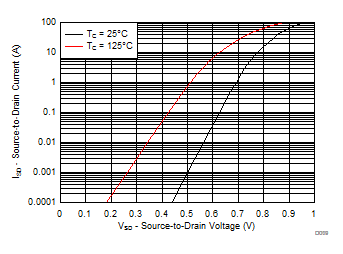Figure 9. Typical Diode Forward Voltage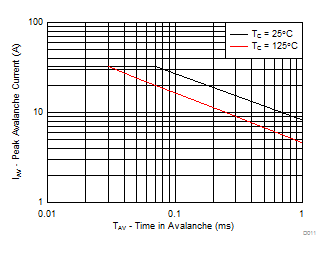Figure 11. Single Pulse Unclamped Inductive Switching